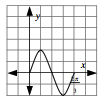### Home > CALC > Chapter 1 > Lesson 1.4.3 > Problem1-174

1-174.

A focus of this course will be determining maximum and minimum values for a function on a given interval. However, you already have the skills to do this for certain functions. On your paper, sketch $y = 2\text{sin}(3x)$ for $0 \leq x \leq \frac { 2 \pi } { 3 }$. Find the points at which $y$ is a maximum and minimum.

See graph below.It is a sine curve with amplitude $2$ and period $\frac{2\pi}{3}$.

The graph is a sine curve that is vertically stretched by a factor of $2$ and horizontally compressed by a factor of $3$.

Maximum at $(\frac{\pi}{6},?)$, minimum at $(?,-2)$.